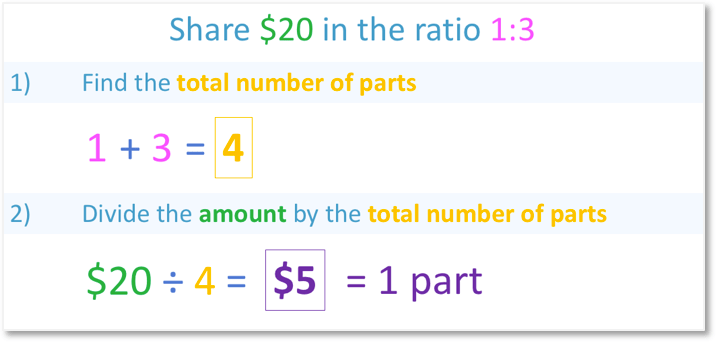# How to Calculate a Ratio of a Number• In this example, we are sharing £50 in the ratio 2:3, using steps.

• Step 1 is to find the total number of parts in our ratio.

• 2 + 3 = 5. Our ratio of 2:3 contains 5 parts in total.

• Step 2 is to find the value of one part by dividing the amount by the total number of parts.

• £50 ÷ 5 = £10.

• Step 3 is to scale up the original ratio by the value of one part of the ratio.

• 2:3 becomes £20:£30 when we multiply by £10.

• One person gets £20 and the other gets £30. These values add to our total of £50.

To calculate the ratio of an amount we divide the amount by the total number of parts in the ratio and then multiply this answer by the original ratio.• We want to work out $20 shared in the ratio of 1:3. • Step 1 is to work out the total number of parts in the ratio. • 1 + 3 = 4, so the ratio 1:3 contains 4 parts in total. • Step 2 is to divide the amount by the total number of parts in the ratio. •$20 ÷ 4 = $5. • Each of the four parts of the ratio is worth$5.

• Step 3 is to scale up our original ratio by the value of each part.

• 1:3 multiplied by $5 becomes$5:$15. • One person gets$5 and the other gets $15. • We can check our ratio calculation by adding$5 and $15 to get our total amount of$20.Supporting Lessons# How to Calculate a Ratio of a Number

## What is a Ratio?

Ratios describe how to share out a given amount and are written with numbers separated by colons. The amount of numbers in the ratio tells us how many groups the quantity is being shared between. The size of each number tells us the proportion of the total amount each group gets.

For example, in the ratio 1:3 there are two different numbers: ‘1’ and ‘3’.

Because there are two numbers, we are sharing an amount between two people.

This ratio means that for every 1 part that the person on the left gets, the person on the right gets 3. The person on the right will have three times as much as the person on the left.The person on the left will get $5 and the person on the right will get$15. Both numbers add to make the total of $20 but$15 is three times larger than $5. This is why the ratio is 1 to 3. ## How to Calculate a Ratio of a Number in Steps To calculate a ratio of a number, follow these 3 steps: 1. Add the parts of the ratio to find the total number of parts. 2. Find the value of each part of the ratio by dividing the number by the total number of parts calculated in step 1. 3. Multiply each part of the original ratio by the value of each part calculated in step 2. For example, share$20 in the ratio 1:3.This means that in this ratio problem, we will be sharing $20 between two people with one person getting three times as much as the other. We follow the 3 steps above to work out the ratio. • Step 1: Find the total number of partsLooking at the ratio 1:3, we have: 1 + 3 = 4 So, we have four parts in total in our ratio. • Step 2: Divide the amount by the total number of partsThe amount is$20 and the total number of parts is 4.

20 ÷ 4 = 5

Each of the four parts is worth $5. • Step 3: Multiply each number in the ratio by the value of one partWe have four parts in total and each of these parts are worth$5.

We will multiply both numbers in the ratio 1:3 by $5. 1 x$5 = $5 3 x$5 = $15 The money is shared in the ratio$5:$15. We have solved our ratio problem to find that one person gets$5 and the other person gets $15. To check a ratio calculation, add the final values to see if the total is equal to the original amount. If the amounts shared can also be simplified to make the ratio given, the calculation is correct.$5 + $15 =$20, which is the amount that we started with, so we can be confident that our calculation is correct.

To confirm our ratio calculation, we check that $5:$15 simplifies to give us our original ratio.

Dividing both $5 and$15 by 5, gives us 1:3, which is our original ratio and $15 is indeed three times as much as$5.Now try our lesson on Sharing in a Ratio Between 3 People where we learn how to extend our steps for calculating ratio to examples where we share an amount between 3 people.error: Content is protected !!